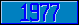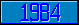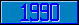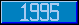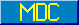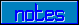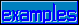## Collation Algorithm

### Collation Algorithm:

^\$Global( gvnexpr , expr V "COLLATE" ) = expr V algoref

This node identifies the collation algorithm to be used when collation is required for a reference to this global. The collation value order for a subscript-string subscript, and a collation algorithm collate may be determined by executing the expression: ("S order="_collate_"(subscript)"). In all cases a collation algorithm must return a distinct order for each distinct subscript.

When the first node of a global global is created, and the collation algorithm node ^\$Global("global","COLLATE") has a \$Data value of zero, then the value of the current process' Character Set Profile collation algorithm ( \$Get(^\$Character(^\$Job(\$Job,"CHARACTER"),"COLLATE")) ) is assigned as the global's collation algorithm ( ^\$Global("global","COLLATE") ).